Updating search results...

# 123 Results

View
Selected filters:
• word-problemsUnrestricted Use
CC BY
Rating
0.0 stars

In this task, students can see that if the price level increases and peopleŐs incomes do not increase, they arenŐt able to purchase as many goods and services; in other words, their purchasing power decreases.

Subject:
Mathematics
Numbers and Operations
Material Type:
Activity/Lab
Provider:
Illustrative Mathematics
Provider Set:
Illustrative Mathematics
Author:
Illustrative Mathematics
12/19/2012Unrestricted Use
CC BY
Rating
0.0 stars

In this task students use different representations to analyze the relationship between two quantities and to solve a real world problem. The situation presented provides a good opportunity to make connections between the information provided by tables, graphs and equations.

Subject:
Mathematics
Material Type:
Activity/Lab
Provider:
Illustrative Mathematics
Provider Set:
Illustrative Mathematics
Author:
Illustrative Mathematics
08/08/2012Unrestricted Use
CC BY
Rating
0.0 stars

The purpose of this task is to foster a classroom discussion that will highlight the difference between multiplicative and additive reasoning.

Subject:
Mathematics
Numbers and Operations
Material Type:
Activity/Lab
Provider:
Illustrative Mathematics
Provider Set:
Illustrative Mathematics
Author:
Illustrative Mathematics
05/01/2012Unrestricted Use
CC BY
Rating
0.0 stars

The purpose of this task is to assess studentsŐ understanding of multiplicative and additive reasoning.

Subject:
Mathematics
Numbers and Operations
Material Type:
Activity/Lab
Provider:
Illustrative Mathematics
Provider Set:
Illustrative Mathematics
Author:
Illustrative Mathematics
Institute for Mathematics & Education funded by the Bill & Melinda Gates Foundation
05/01/2012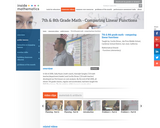Rating
0.0 stars

The foundation of this lesson is constructing, communicating, and evaluating student-generated tables while making comparisons between three different financial plans. Students are given three different DVD rental plans and asked to analyze each one to see if they could determine when the 3 different DVD plans cost the same amount of money, if ever. (7th/8th Grade Math)

Subject:
Algebra
Mathematics
Material Type:
Activity/Lab
Lecture
Lesson Plan
Teaching/Learning Strategy
Provider:
Noyce Foundation
Provider Set:
Inside Mathematics
Author:
Dimas, Cecilio
11/30/2011Some Rights Reserved
Rating
0.0 stars

In these lessons students will explore the paintings of Horace Pippin and Wayne Thiebaud and the mobiles of Alexander Calder to discover and practice math and visual art concepts. Background and biographical information about the work of art and artist, guided looking with class discussion, and activities with worksheets using mathematical formulas and studio art provide the framework for each lesson.

Subject:
Arts and Humanities
Geometry
Mathematics
Visual Arts
Material Type:
Activity/Lab
Assessment
Lesson Plan
Provider:
National Gallery of Art
02/16/2011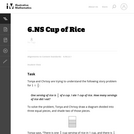Unrestricted Use
CC BY
Rating
0.0 stars

One common mistake students make when dividing fractions using visuals is the confusion between remainder and the fractional part of a mixed number answer.

Subject:
Mathematics
Material Type:
Activity/Lab
Provider:
Illustrative Mathematics
Provider Set:
Illustrative Mathematics
Author:
Illustrative Mathematics
05/01/2012Unrestricted Use
CC BY
Rating
0.0 stars

Students must convert yards to feet to solve this word problem.

Subject:
Mathematics
Material Type:
Activity/Lab
Provider:
Illustrative Mathematics
Provider Set:
Illustrative Mathematics
Author:
Illustrative Mathematics
05/01/2012Conditional Remix & Share Permitted
CC BY-NC
Rating
0.0 stars

Students solve decimal multiplication and division problems related to the basic fact 3 &times; 7 = 21.Students match cards that represent word problems, visual models, and numerical solutions to problems that include the numbers 0.8 and 0.2 for all four operations.Key ConceptsNo new mathematics is introduced in this lesson. Students apply their knowledge about decimal operations.Goals and Learning ObjectivesUse reasoning and mental math to solve problems.Solve word problems involving simple addition, subtraction, multiplication, and division with decimals.

Subject:
Mathematics
Author:
April Hamma
06/09/2022Unrestricted Use
CC BY
Rating
0.0 stars

This purpose of this task is to help students see two different ways to look at percentages both as a decrease and an increase of an original amount. In addition, students have to turn a verbal description of several operations into mathematical symbols.

Subject:
Algebra
Mathematics
Material Type:
Activity/Lab
Provider:
Illustrative Mathematics
Provider Set:
Illustrative Mathematics
Author:
Illustrative Mathematics
05/01/2012Unrestricted Use
CC BY
Rating
0.0 stars

This task asks students to find equivalent expressions by visualizing a familiar activity involving distance. The given solution shows some possible equivalent expressions, but there are many variations possible.

Subject:
Mathematics
Material Type:
Activity/Lab
Provider:
Illustrative Mathematics
Provider Set:
Illustrative Mathematics
Author:
Illustrative Mathematics
05/01/2012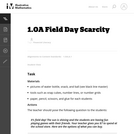Unrestricted Use
CC BY
Rating
0.0 stars

This task allows students to relate addition and subtraction problems to money in a context that introduces the concept of scarcity.

Subject:
Mathematics
Numbers and Operations
Material Type:
Activity/Lab
Provider:
Illustrative Mathematics
Provider Set:
Illustrative Mathematics
Author:
Illustrative Mathematics
02/10/2013Unrestricted Use
CC BY
Rating
0.0 stars

In this task students are asked to write an equation to solve a real-world problem. There are two natural approaches to this task. In the first approach, students have to notice that even though there is one variable, namely the number of firefighters, it is used in two different places. In the other approach, students can find the total cost per firefighter and then write the equation.

Subject:
Mathematics
Material Type:
Activity/Lab
Provider:
Illustrative Mathematics
Provider Set:
Illustrative Mathematics
Author:
Illustrative Mathematics
05/01/2012Unrestricted Use
CC BY
Rating
0.0 stars

This task is the first in a series of three tasks that use inequalities in the same context at increasing complexity in 6th grade, 7th grade and in HS algebra. Students write and solve inequalities, and represent the solutions graphically.

Subject:
Mathematics
Material Type:
Activity/Lab
Provider:
Illustrative Mathematics
Provider Set:
Illustrative Mathematics
Author:
Illustrative Mathematics
05/01/2012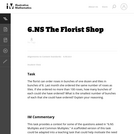Unrestricted Use
CC BY
Rating
0.0 stars

This task provides a context for some of the questions asked in "6.NS Multiples and Common Multiples." A scaffolded version of this task could be adapted into a teaching task that could help motivate the need for the concept of a common multiple.

Subject:
Mathematics
Material Type:
Activity/Lab
Provider:
Illustrative Mathematics
Provider Set:
Illustrative Mathematics
Author:
Illustrative Mathematics
05/01/2012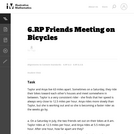Unrestricted Use
CC BY
Rating
0.0 stars

This series of 5 word problems lead up to the final problem. Most students should be able to answer the first two questions without too much difficulty. The decimal numbers may cause some students trouble, but if they make a drawing of the road that the girls are riding on, and their positions at the different times, it may help. The third question has a bit of a challenge in that students won't land on the exact meeting time by making a table with distance values every hour. The fourth question addresses a useful concept for problems involving objects moving at different speeds which may be new to sixth grade students.

Subject:
Mathematics
Material Type:
Activity/Lab
Provider:
Illustrative Mathematics
Provider Set:
Illustrative Mathematics
Author:
Illustrative Mathematics
05/01/2012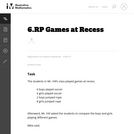Unrestricted Use
CC BY
Rating
0.0 stars

While students need to be able to write sentences describing ratio relationships, they also need to see and use the appropriate symbolic notation for ratios. If this is used as a teaching problem, the teacher could ask for the sentences as shown, and then segue into teaching the notation. It is a good idea to ask students to write it both ways (as shown in the solution) at some point as well.

Subject:
Mathematics
Material Type:
Activity/Lab
Provider:
Illustrative Mathematics
Provider Set:
Illustrative Mathematics
Author:
Illustrative Mathematics